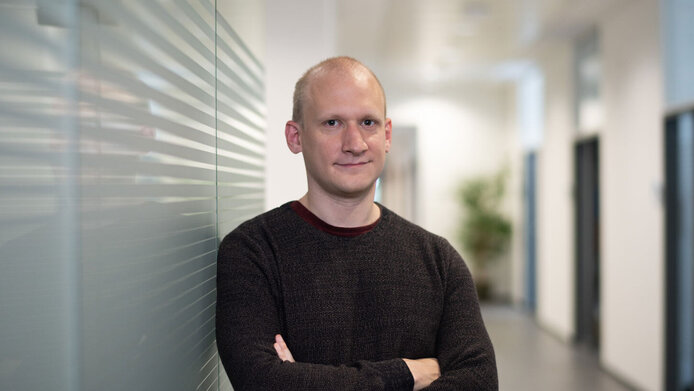The mathematical foundations of many theories are often not fully understood. Mathematician Máté Gerencsér is focusing on fundamentally understanding models and translating them into applications. © FWF/Daniel Novotny

Máté Gerencsér: We are looking at certain mathematical equations called stochastic partial differential equations, abbreviated as SPDEs, and their solutions. These differential equations typically describe processes that change over time, like how heat spreads through an object. A solution to such a partial differential equation is not a single number – like finding x in the equation 2x=10 – but a function of the variables involved. The stochastic part means that we introduce some noise into the partial differential equations. Noise is small random disturbances in the equation that then affect the functions that are the equation’s solutions.

What answers are you aiming to find?

Gerencsér: Mathematicians often want to know the long-term behavior of the solutions of these kinds of equations. This means that on short time scales, the function may behave in one way – its value may go up or down or behave erratically – but after a sufficiently long time, it will settle into a kind of characteristic, stable behavior. For example, when you heat one end of a bar of metal, the heat will be distributed evenly in the whole bar after a long enough time. The interesting long-time random patterns that SPDEs produce are among the main motivations to study them.

Máté Gerencsér works as an associate professor for “Numerics of stochastic differential equations” at the Institute of Analysis and Scientific Computing at the TU Wien. He first studied mathematics in Hungary at the Eötvös Loránd University in Budapest before receiving his PhD from the University of Edinburgh in 2016. After a brief stay at the University of Warwick, UK, he worked as a postdoc at the Institute of Science and Technology Austria (ISTA).

“Mathematicians often want to know the long-term behavior of the solutions of these kinds of equations.” Máté Gerencsér

While the added noise helps us find solutions in some stochastic partial differential equations, it can make it harder in others. In my research, I am looking at these equations and want to answer two kinds of questions: First, how can we use mathematical tools to better understand these equations and create a rigorous mathematical foundation explaining their behavior? And second, how can we effectively implement these equations in computational simulations?

Why is this research important?

Gerencsér: Stochastic partial differential equations appear in various fields, from statistical mechanics to population genetics to polymer physics. In physics or chemistry, there are often mathematical structures that are thought to be well-understood, but it can take a long time for mathematics to fully describe them and to create the rigorous mathematical underpinnings needed to prove their fundamental properties. For example, this is the case in quantum field theory, which is an extremely successful theory, but its mathematics are far from understood. There, we could apply our work to better understand some specific models.

What initial steps do you plan to take to answer these questions?

Gerencsér: When studying differential equations, we usually think about finding functions as their solutions. However, for many stochastic partial differential equations, there is a more fundamental challenge. We first have to make sense of the equations themselves and understand all their terms and how they interact.

“The fundamental challenge is to make sense of the equations and all their terms themselves. ” Máté Gerencsér

These equations can also show some strange behavior. Certain terms in them can become infinitely large, which cannot directly correspond to any feature of the real world these equations try to model. As one of our first steps, we need to deal with these infinities using a mathematical technique called renormalization.

What will the START Award mean for your research activities?

Gerencsér: This award is a great opportunity to focus on these questions and to create a new research group that can tackle these difficult and time-consuming problems. We will have the chance to study these questions in depth and from a number of different angles.

What motivates you in your day-to-day research?

Gerencsér: I am just interested in exploring the beauty of mathematics. Sometimes I think mathematics is as much art as science. The creativity involved and the ability to prove something definitively are both very attractive to me.

What role models have inspired you?

Gerencsér: I had many great teachers during my studies in Hungary. A big influence was my PhD advisor in Edinburgh, István Gyöngy. I was also very lucky in that during my PhD, my field of studies in mathematics was revolutionized by the work of the Austrian mathematician Martin Hairer and I had the chance to work with him to see what such a major breakthrough looks like close-up.

### The FWF START Award

The START program of the Austrian Science Fund (FWF) is aimed at outstanding young researchers, giving them the opportunity to plan their research in the long term and with a high degree of financial security. It is endowed with up to €1.2 million and is one of Austria’s most prestigious and most highly endowed awards alongside the FWF Wittgenstein Award.